# Selina Solutions Concise Maths Class 10 Chapter 3 Shares and Dividends Exercise 3(C)

Having understood the basics of shares and dividends students will now understand the different choices of investment and other comparisons. The exercise problems are well framed to give a thorough understanding of shares and dividends. Students can make use of the Selina Solutions for Class 10 Maths to acquire a strong grip in solving such problems. Also, access the Concise Selina Solutions for Class 10 Maths Chapter 3 Shares and Dividends Exercise 3(C) PDF that available below.

## Selina Solutions Concise Maths Class 10 Chapter 3 Shares and Dividends Exercise 3(C) Download PDF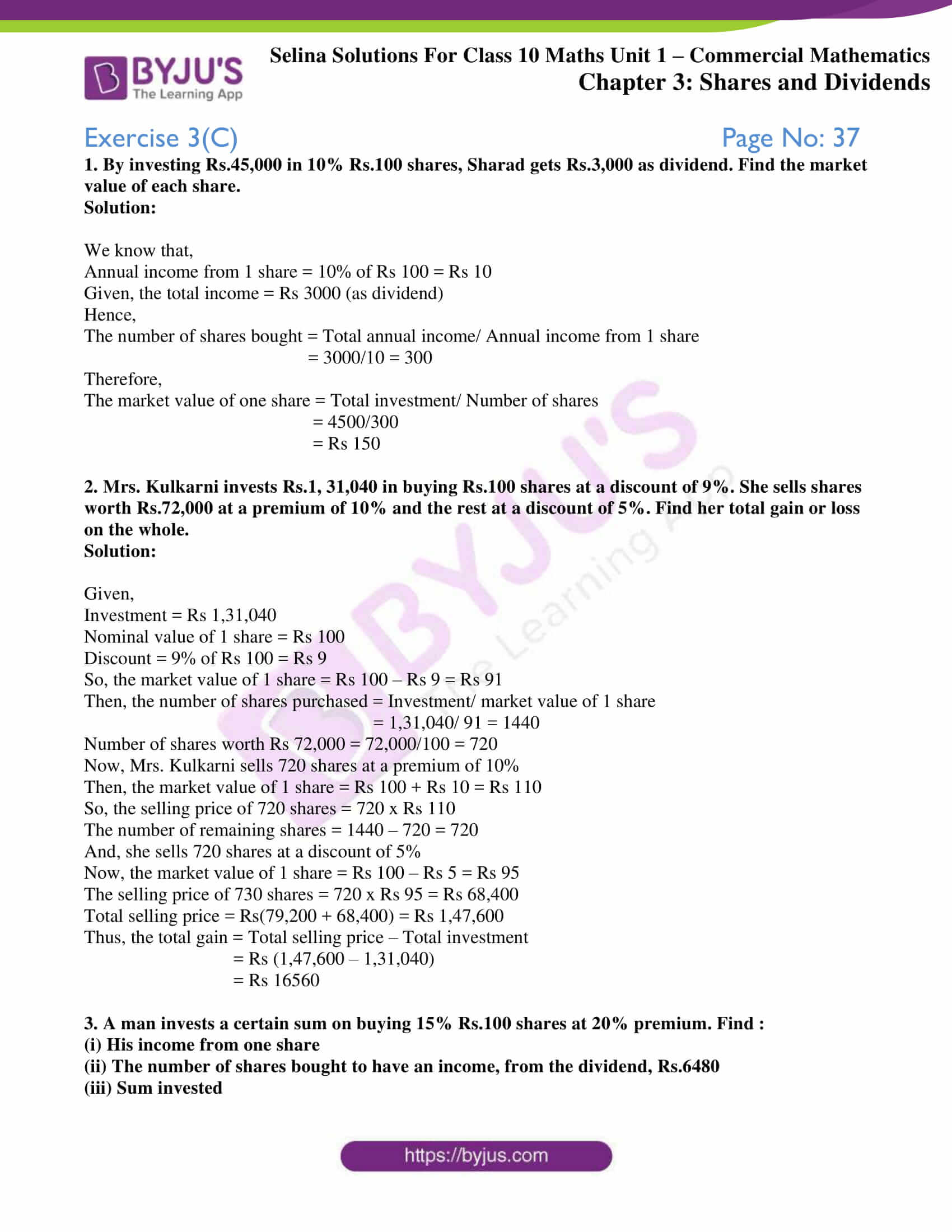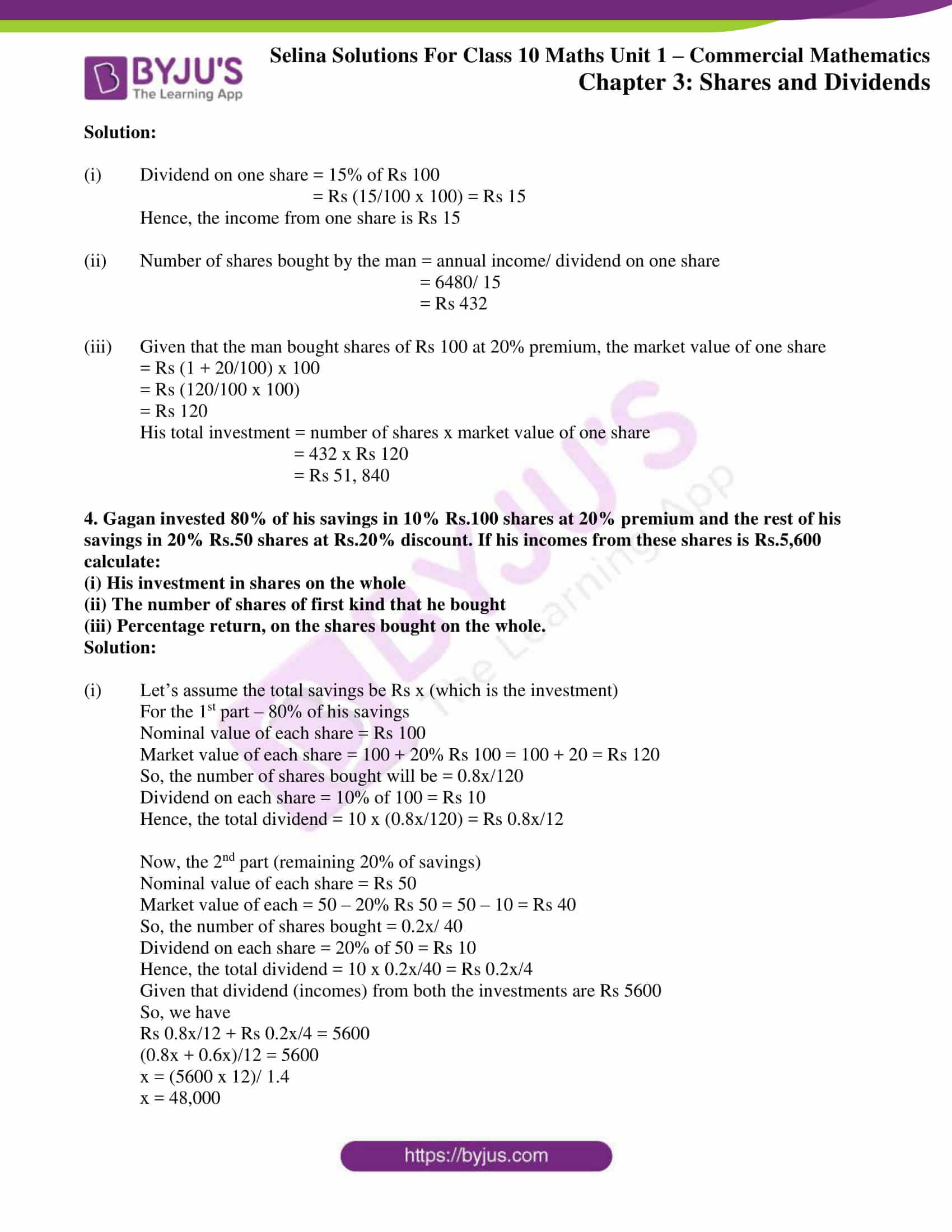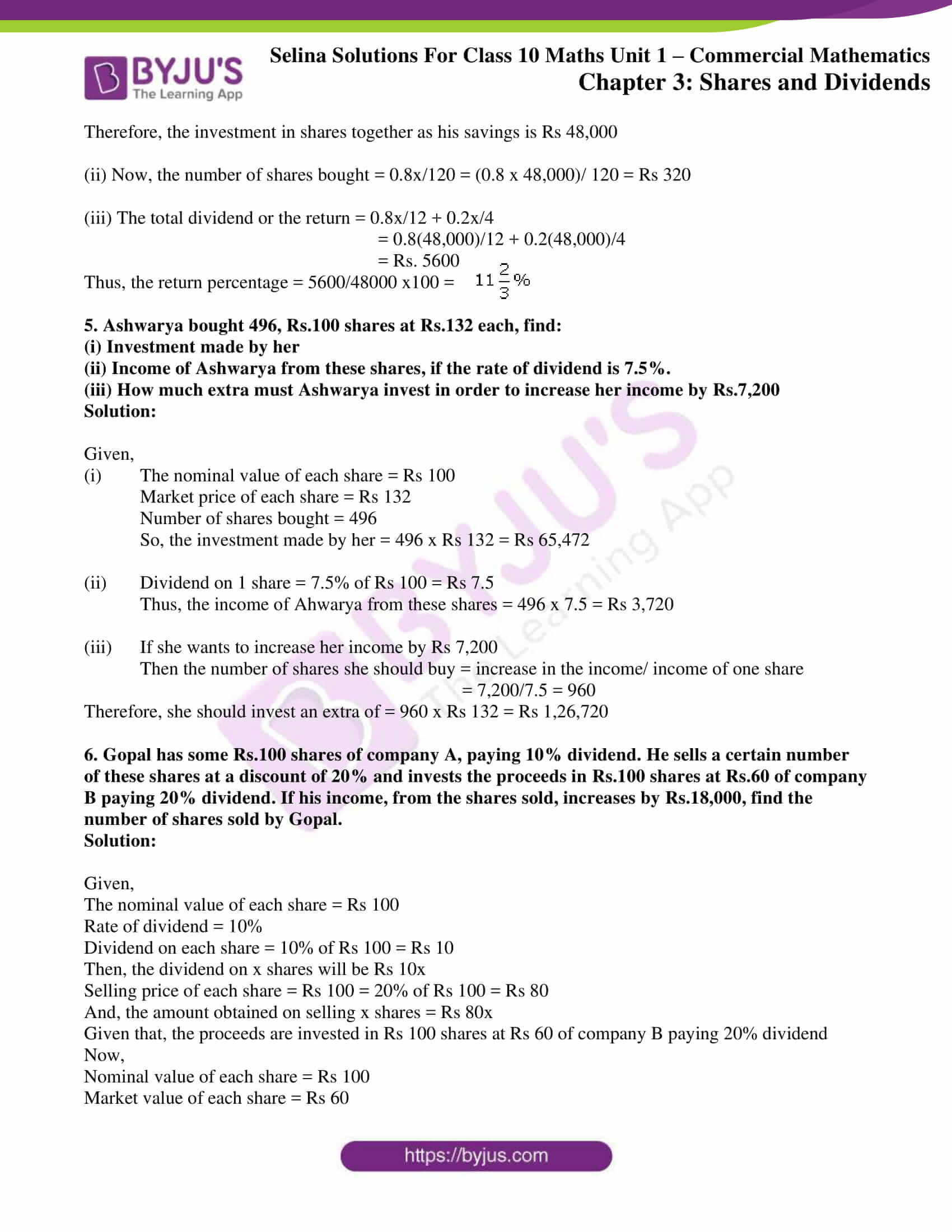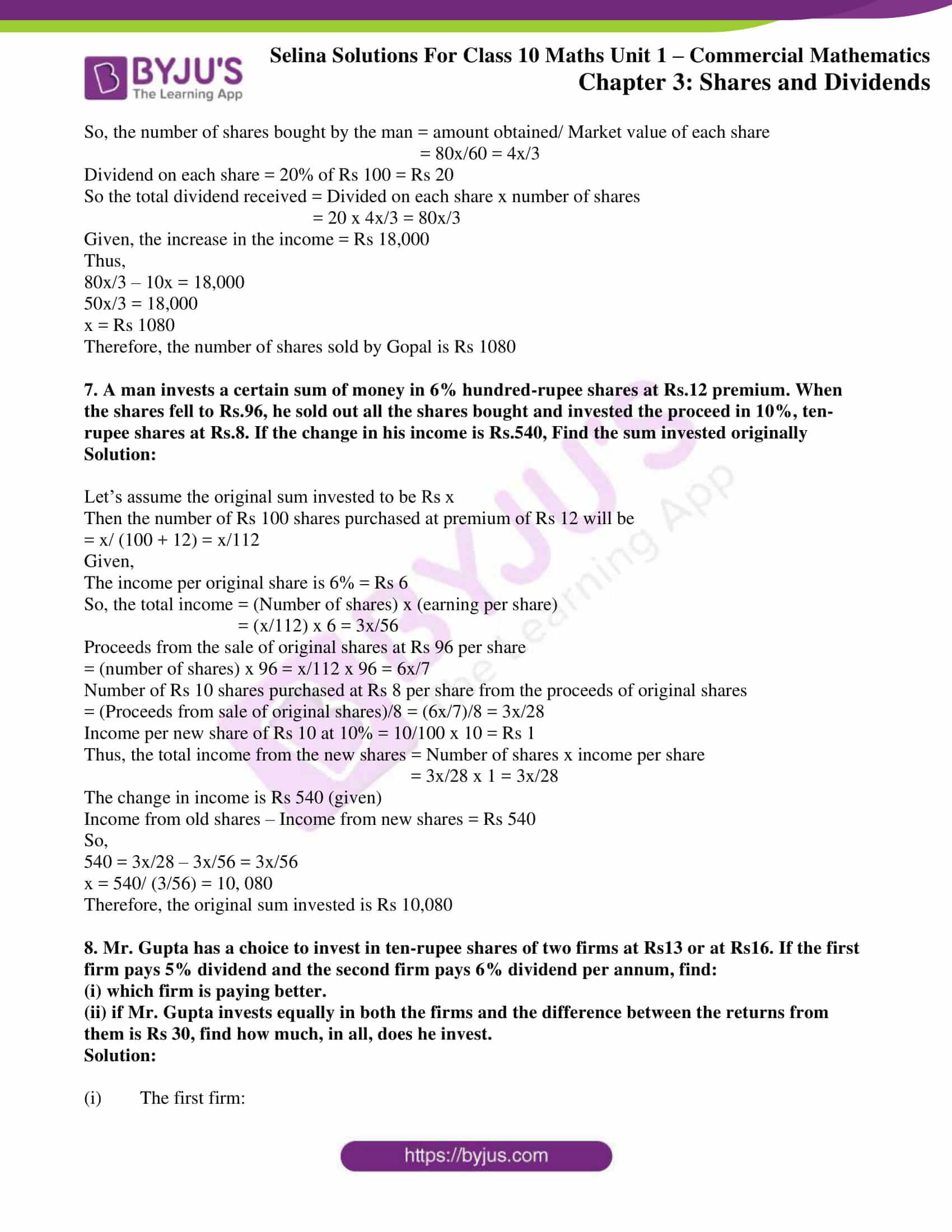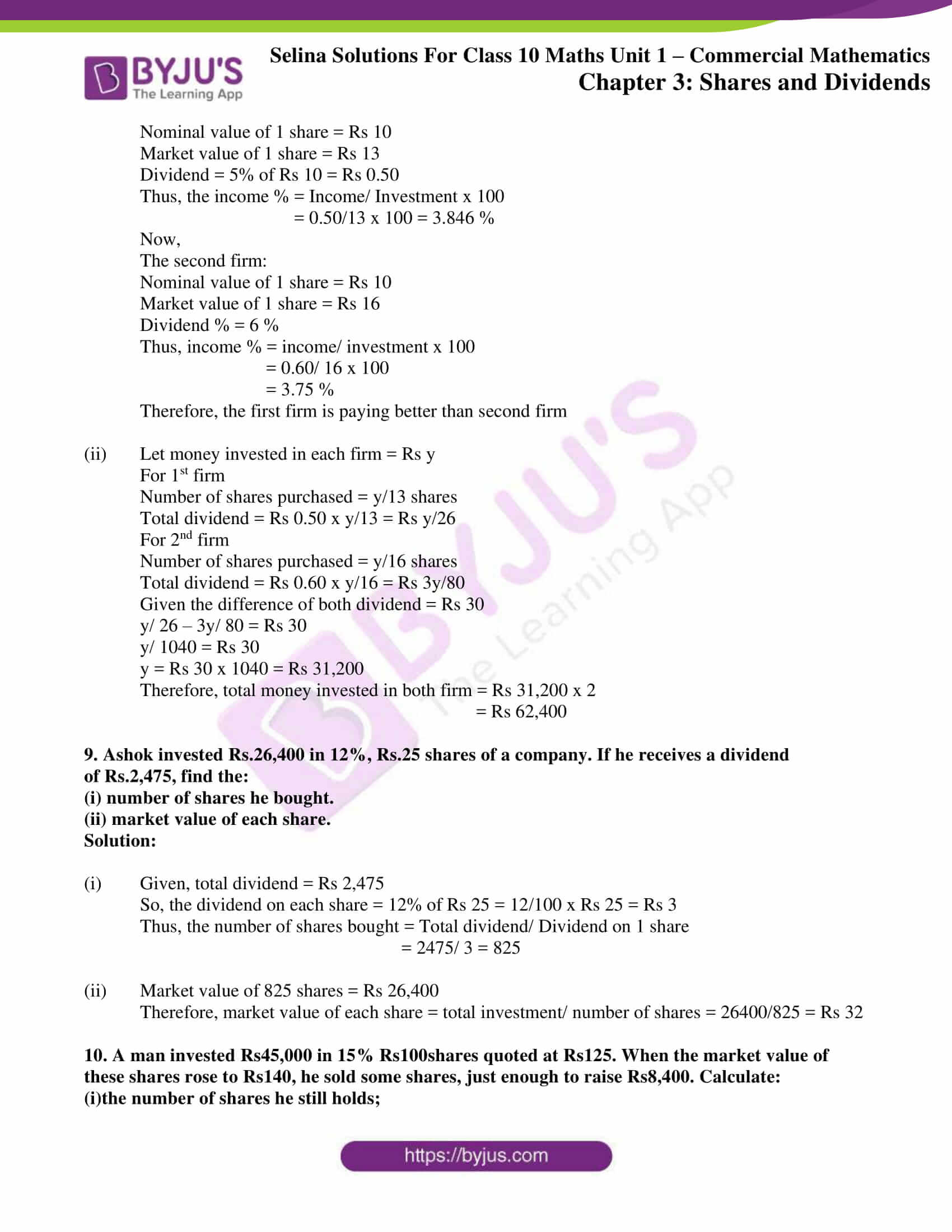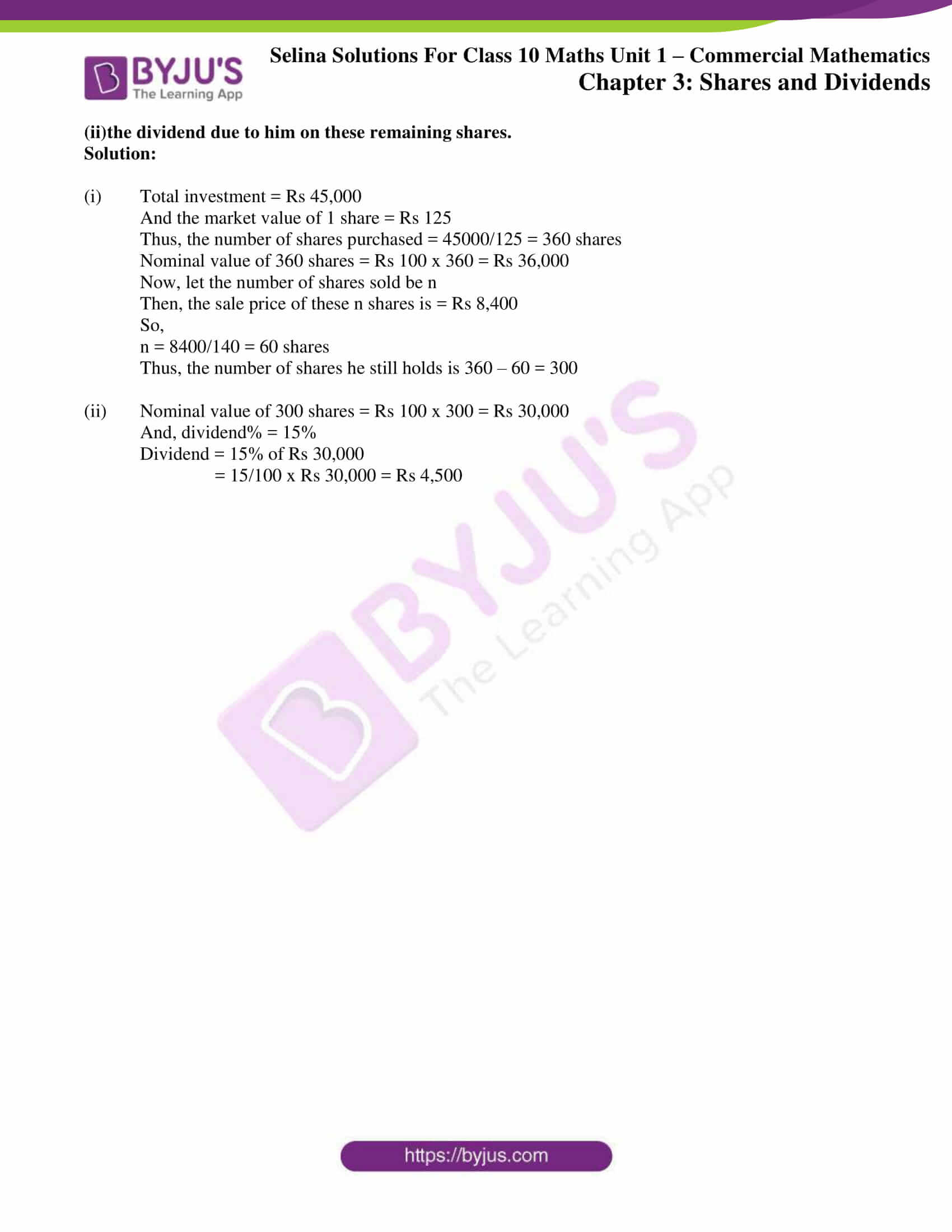### Access Selina Solutions Concise Maths Class 10 Chapter 3 Shares and Dividends Exercise 3(C)

1. By investing Rs.45,000 in 10% Rs.100 shares, Sharad gets Rs.3,000 as dividend. Find the market value of each share.

Solution:

We know that,

Annual income from 1 share = 10% of Rs 100 = Rs 10

Given, the total income = Rs 3000 (as dividend)

Hence,

The number of shares bought = Total annual income/ Annual income from 1 share

= 3000/10 = 300

Therefore,

The market value of one share = Total investment/ Number of shares

= 4500/300

= Rs 150

2. Mrs. Kulkarni invests Rs.1, 31,040 in buying Rs.100 shares at a discount of 9%. She sells shares worth Rs.72,000 at a premium of 10% and the rest at a discount of 5%. Find her total gain or loss on the whole.

Solution:

Given,

Investment = Rs 1,31,040

Nominal value of 1 share = Rs 100

Discount = 9% of Rs 100 = Rs 9

So, the market value of 1 share = Rs 100 – Rs 9 = Rs 91

Then, the number of shares purchased = Investment/ market value of 1 share

= 1,31,040/ 91 = 1440

Number of shares worth Rs 72,000 = 72,000/100 = 720

Now, Mrs. Kulkarni sells 720 shares at a premium of 10%

Then, the market value of 1 share = Rs 100 + Rs 10 = Rs 110

So, the selling price of 720 shares = 720 x Rs 110

The number of remaining shares = 1440 – 720 = 720

And, she sells 720 shares at a discount of 5%

Now, the market value of 1 share = Rs 100 – Rs 5 = Rs 95

The selling price of 730 shares = 720 x Rs 95 = Rs 68,400

Total selling price = Rs(79,200 + 68,400) = Rs 1,47,600

Thus, the total gain = Total selling price – Total investment

= Rs (1,47,600 – 1,31,040)

= Rs 16560

3. A man invests a certain sum on buying 15% Rs.100 shares at 20% premium. Find :

(i) His income from one share

(ii) The number of shares bought to have an income, from the dividend, Rs.6480

(iii) Sum invested

Solution:

(i) Dividend on one share = 15% of Rs 100

= Rs (15/100 x 100) = Rs 15

Hence, the income from one share is Rs 15

(ii) Number of shares bought by the man = annual income/ dividend on one share

= 6480/ 15

= Rs 432

(iii) Given that the man bought shares of Rs 100 at 20% premium, the market value of one share

= Rs (1 + 20/100) x 100

= Rs (120/100 x 100)

= Rs 120

His total investment = number of shares x market value of one share

= 432 x Rs 120

= Rs 51, 840

4. Gagan invested 80% of his savings in 10% Rs.100 shares at 20% premium and the rest of his savings in 20% Rs.50 shares at Rs.20% discount. If his incomes from these shares is Rs.5,600 calculate:

(i) His investment in shares on the whole

(ii) The number of shares of first kind that he bought

(iii) Percentage return, on the shares bought on the whole.

Solution:

(i) Let’s assume the total savings be Rs x (which is the investment)

For the 1st part – 80% of his savings

Nominal value of each share = Rs 100

Market value of each share = 100 + 20% Rs 100 = 100 + 20 = Rs 120

So, the number of shares bought will be = 0.8x/120

Dividend on each share = 10% of 100 = Rs 10

Hence, the total dividend = 10 x (0.8x/120) = Rs 0.8x/12

Now, the 2nd part (remaining 20% of savings)

Nominal value of each share = Rs 50

Market value of each = 50 – 20% Rs 50 = 50 – 10 = Rs 40

So, the number of shares bought = 0.2x/ 40

Dividend on each share = 20% of 50 = Rs 10

Hence, the total dividend = 10 x 0.2x/40 = Rs 0.2x/4

Given that dividend (incomes) from both the investments are Rs 5600

So, we have

Rs 0.8x/12 + Rs 0.2x/4 = 5600

(0.8x + 0.6x)/12 = 5600

x = (5600 x 12)/ 1.4

x = 48,000

Therefore, the investment in shares together as his savings is Rs 48,000

(ii) Now, the number of shares bought = 0.8x/120 = (0.8 x 48,000)/ 120 = Rs 320

(iii) The total dividend or the return = 0.8x/12 + 0.2x/4

= 0.8(48,000)/12 + 0.2(48,000)/4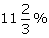= Rs. 5600

Thus, the return percentage = 5600/48000 x100 =

5. Ashwarya bought 496, Rs.100 shares at Rs.132 each, find:

(ii) Income of Ashwarya from these shares, if the rate of dividend is 7.5%.

(iii) How much extra must Ashwarya invest in order to increase her income by Rs.7,200

Solution:

Given,

(i) The nominal value of each share = Rs 100

Market price of each share = Rs 132

Number of shares bought = 496

So, the investment made by her = 496 x Rs 132 = Rs 65,472

(ii) Dividend on 1 share = 7.5% of Rs 100 = Rs 7.5

Thus, the income of Ahwarya from these shares = 496 x 7.5 = Rs 3,720

(iii) If she wants to increase her income by Rs 7,200

Then the number of shares she should buy = increase in the income/ income of one share

= 7,200/7.5 = 960

Therefore, she should invest an extra of = 960 x Rs 132 = Rs 1,26,720

6. Gopal has some Rs.100 shares of company A, paying 10% dividend. He sells a certain number of these shares at a discount of 20% and invests the proceeds in Rs.100 shares at Rs.60 of company B paying 20% dividend. If his income, from the shares sold, increases by Rs.18,000, find the number of shares sold by Gopal.

Solution:

Given,

The nominal value of each share = Rs 100

Rate of dividend = 10%

Dividend on each share = 10% of Rs 100 = Rs 10

Then, the dividend on x shares will be Rs 10x

Selling price of each share = Rs 100 = 20% of Rs 100 = Rs 80

And, the amount obtained on selling x shares = Rs 80x

Given that, the proceeds are invested in Rs 100 shares at Rs 60 of company B paying 20% dividend

Now,

Nominal value of each share = Rs 100

Market value of each share = Rs 60

So, the number of shares bought by the man = amount obtained/ Market value of each share

= 80x/60 = 4x/3

Dividend on each share = 20% of Rs 100 = Rs 20

So the total dividend received = Divided on each share x number of shares

= 20 x 4x/3 = 80x/3

Given, the increase in the income = Rs 18,000

Thus,

80x/3 – 10x = 18,000

50x/3 = 18,000

x = Rs 1080

Therefore, the number of shares sold by Gopal is Rs 1080

7. A man invests a certain sum of money in 6% hundred-rupee shares at Rs.12 premium. When the shares fell to Rs.96, he sold out all the shares bought and invested the proceed in 10%, ten-rupee shares at Rs.8. If the change in his income is Rs.540, Find the sum invested originally

Solution:

Let’s assume the original sum invested to be Rs x

Then the number of Rs 100 shares purchased at premium of Rs 12 will be

= x/ (100 + 12) = x/112

Given,

The income per original share is 6% = Rs 6

So, the total income = (Number of shares) x (earning per share)

= (x/112) x 6 = 3x/56

Proceeds from the sale of original shares at Rs 96 per share

= (number of shares) x 96 = x/112 x 96 = 6x/7

Number of Rs 10 shares purchased at Rs 8 per share from the proceeds of original shares

= (Proceeds from sale of original shares)/8 = (6x/7)/8 = 3x/28

Income per new share of Rs 10 at 10% = 10/100 x 10 = Rs 1

Thus, the total income from the new shares = Number of shares x income per share

= 3x/28 x 1 = 3x/28

The change in income is Rs 540 (given)

Income from old shares – Income from new shares = Rs 540

So,

540 = 3x/28 – 3x/56 = 3x/56

x = 540/ (3/56) = 10, 080

Therefore, the original sum invested is Rs 10,080

8. Mr. Gupta has a choice to invest in ten-rupee shares of two firms at Rs13 or at Rs16. If the first firm pays 5% dividend and the second firm pays 6% dividend per annum, find:

(i) which firm is paying better.

(ii) if Mr. Gupta invests equally in both the firms and the difference between the returns from them is Rs 30, find how much, in all, does he invest.

Solution:

(i) The first firm:

Nominal value of 1 share = Rs 10

Market value of 1 share = Rs 13

Dividend = 5% of Rs 10 = Rs 0.50

Thus, the income % = Income/ Investment x 100

= 0.50/13 x 100 = 3.846 %

Now,

The second firm:

Nominal value of 1 share = Rs 10

Market value of 1 share = Rs 16

Dividend % = 6 %

Thus, income % = income/ investment x 100

= 0.60/ 16 x 100

= 3.75 %

Therefore, the first firm is paying better than second firm

(ii) Let money invested in each firm = Rs y

For 1st firm

Number of shares purchased = y/13 shares

Total dividend = Rs 0.50 x y/13 = Rs y/26

For 2nd firm

Number of shares purchased = y/16 shares

Total dividend = Rs 0.60 x y/16 = Rs 3y/80

Given the difference of both dividend = Rs 30

y/ 26 – 3y/ 80 = Rs 30

y/ 1040 = Rs 30

y = Rs 30 x 1040 = Rs 31,200

Therefore, total money invested in both firm = Rs 31,200 x 2

= Rs 62,400

9. Ashok invested Rs.26,400 in 12%, Rs.25 shares of a company. If he receives a dividend of Rs.2,475, find the:

(i) number of shares he bought.

(ii) market value of each share.

Solution:

(i) Given, total dividend = Rs 2,475

So, the dividend on each share = 12% of Rs 25 = 12/100 x Rs 25 = Rs 3

Thus, the number of shares bought = Total dividend/ Dividend on 1 share

= 2475/ 3 = 825

(ii) Market value of 825 shares = Rs 26,400

Therefore, market value of each share = total investment/ number of shares = 26400/825 = Rs 32

10. A man invested Rs45,000 in 15% Rs100shares quoted at Rs125. When the market value of these shares rose to Rs140, he sold some shares, just enough to raise Rs8,400. Calculate:

(i)the number of shares he still holds;

(ii)the dividend due to him on these remaining shares.

Solution:

(i) Total investment = Rs 45,000

And the market value of 1 share = Rs 125

Thus, the number of shares purchased = 45000/125 = 360 shares

Nominal value of 360 shares = Rs 100 x 360 = Rs 36,000

Now, let the number of shares sold be n

Then, the sale price of these n shares is = Rs 8,400

So,

n = 8400/140 = 60 shares

Thus, the number of shares he still holds is 360 – 60 = 300

(ii) Nominal value of 300 shares = Rs 100 x 300 = Rs 30,000

And, dividend% = 15%

Dividend = 15% of Rs 30,000

= 15/100 x Rs 30,000 = Rs 4,500